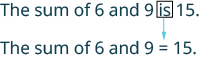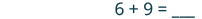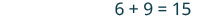Translating Words Involving Addition and Subtraction Into an Algebraic Equation and Solving

Learning Outcomes

• Translate statements into algebraic equations involving addition, subtraction, multiplication, and division

Translate Word Phrases to Algebraic Equations

Remember, an equation has an equal sign between two algebraic expressions. So if we have a sentence that tells us that two phrases are equal, we can translate it into an equation. We look for clue words that mean equals. Some words that translate to the equal sign are:

• is equal to
• is the same as
• is
• gives
• was
• will be

It may be helpful to put a box around the equals word(s) in the sentence to help you focus separately on each phrase. Then translate each phrase into an expression, and write them on each side of the equal sign.

We will practice translating word sentences into algebraic equations. Some of the sentences will be basic number facts with no variables to solve for. Some sentences will translate into equations with variables. The focus right now is just to translate the words into algebra.

example

Translate the sentence into an algebraic equation: The sum of $6$ and $9$ is $15$.

Solution
The word is tells us the equal sign goes between 9 and 15.

 Locate the “equals” word(s).Write the = sign. Translate the words to the left of the equals word into an algebraic expression.Translate the words to the right of the equals word into an algebraic expression.example

Translate the sentence into an algebraic equation: The product of $8$ and $7$ is $56$.

example

Translate the sentence into an algebraic equation: Twice the difference of $x$ and $3$ gives $18$.

Translate to an Equation and Solve

Now let’s practice translating sentences into algebraic equations and then solving them. We will solve the equations by using the Subtraction and Addition Properties of Equality.

example

Translate and solve: Three more than $x$ is equal to $47$.

example

Translate and solve: The difference of $y$ and $14$ is $18$.